#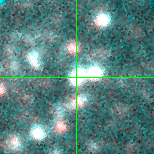UIT026 (J013300.02+303332.4 )

## Target Notes### Classification: -

Three LGGS stars of comparable brightness 2.5--3.0 arcseconds away (J013259.89+303333.5, J013259.91+303334.1, J013259.91+303334.3). Neighbors are blue (comparable brighness in B and V) but not as bright as target in I. Pay heed to blend flag because sometimes resolved. **Changed 180521***

## Plots of Brightness and Color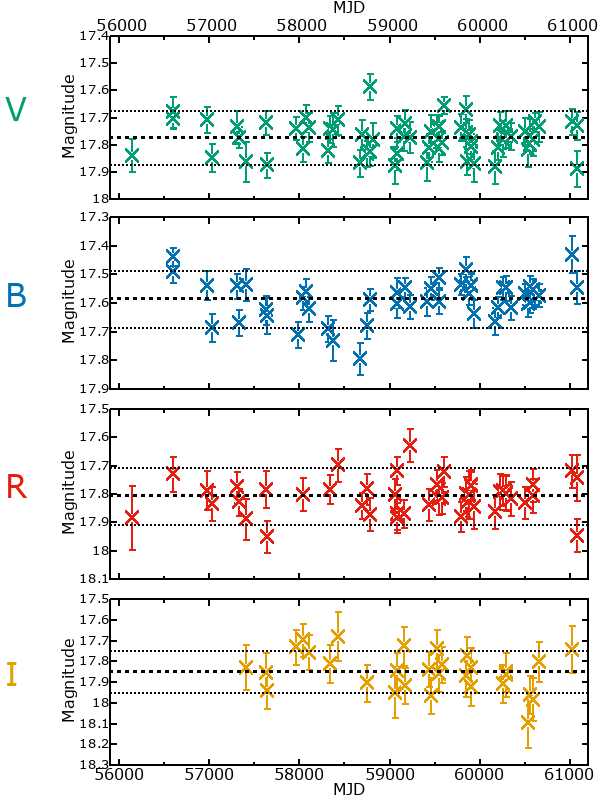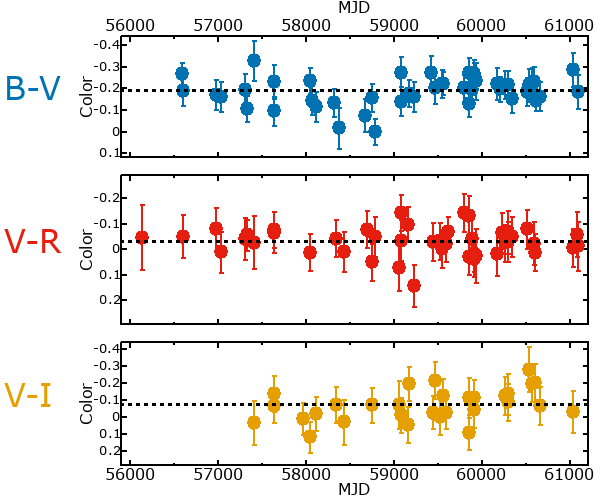## Variability

From the data available in this survey, it is not possible to tell with certainty if this star is variable.

### Correlation factors between bands

If the brightness variation is true variability then there should be a correlation between brightness changes recorded in different filter bands. The following table are the R-squared linear correlation factors calculated for the pairs of bands. The number in parentheses next to the R-squared value is the number of elements used to calculate the correlation factor. The number in square brackets next to the band is the range of magnitudes measured.

V [0.35 mag]B [0.25 mag]R [0.23 mag]
B [0.35 mag]0.23 (21)
R [0.23 mag]0.44 (20)0.10 (13)
I [0.30 mag]0.22 (13)0.01 (8)0.21 (9)

### Magnitude-Magnitude Plots

The plots below are provided to check the values in the table above and also to show any non-linear correlations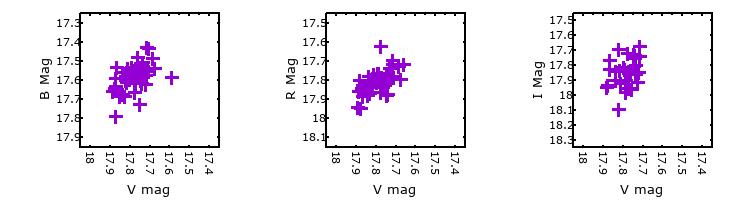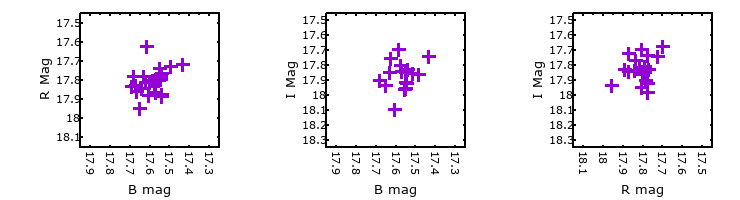### I & R Statistics

Welch & Stetson (1993, AJ, 105, 1813) present a method for varible star detection in CCD images from automated surveys. Data from two bands are compared and two statistics are calculated: R and I.

The I-statistic has an expectation value of zero for stars that are NOT variable. A larger non-zero I-value indicates a higher likelihood that the star is variable. The R statistic indicates how the color of the star changes as the variable grows brighter with R = 1.0 being no change in color.

R and I values have only been calculated if there are more than four (4) matched measurements of brightness in each band.
V
(I|R)
B
(I|R)
R
(I|R)
B
(I|R)
1.38 | 1.26
R
(I|R)
0.71 | 1.230.79 | 0.27
I
(I|R)
0.51 | 1.080.06 | 1.270.59 | 0.90